# Frank solutions for Class 9 Maths ICSE chapter 12 - Isosceles Triangle [Latest edition]

#### Chapters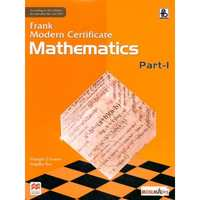## Chapter 12: Isosceles Triangle

Exercise 12.1
Exercise 12.1

### Frank solutions for Class 9 Maths ICSE Chapter 12 Isosceles Triangle Exercise 12.1

Exercise 12.1 | Q 1

Find the angles of an isosceles triangle whose equal angles and the non - equal angles are in the ratio 3: 4.

Exercise 12.1 | Q 2

Find the angles of an isosceles triangle which are in the ratio 2:2:5.

Exercise 12.1 | Q 3

Each equal angle of an isosceles triangle is less than the third angle by 15°. Find the angles.

Exercise 12.1 | Q 4.1

Find the interior angles of the following triangles: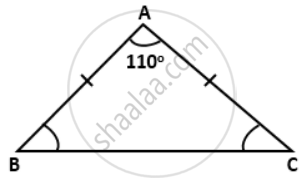Exercise 12.1 | Q 4.2

Find the interior angles of the following triangles: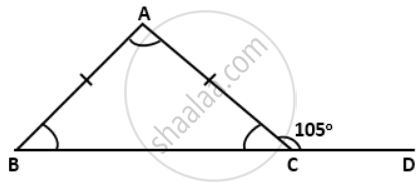Exercise 12.1 | Q 4.3

Find the interior angles of the following triangles: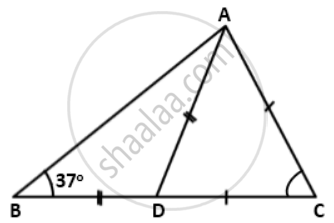Exercise 12.1 | Q 4.4

Find the interior angles of the following triangles: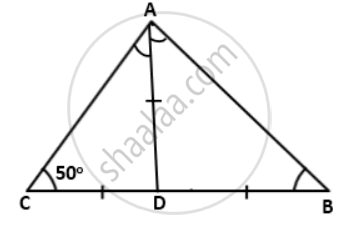Exercise 12.1 | Q 5

Side BA of an isosceles triangle ABC is produced so that AB = AD. If AB and AC are the equal sides of the isosceles triangle, prove that ∠BCD is a right angle.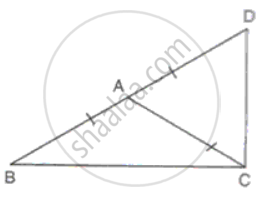Exercise 12.1 | Q 6

The bisector of the equal angles of an isosceles triangle PQR meet at O. If PQ = PR, prove that PO bisects ∠P.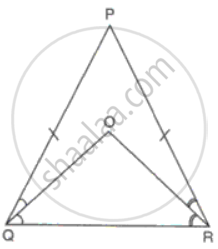Exercise 12.1 | Q 7

Prove that the medians corresponding to equal sides of an isosceles triangle are equal.

Exercise 12.1 | Q 8

DPQ is an isosceles triangle with DP = DQ. A straight line CD bisects the exterior ∠QDR. Prove that DC is parallel to PQ.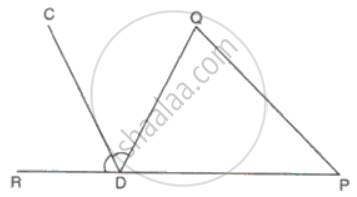Exercise 12.1 | Q 9

In a quadrilateral PQRS, PQ = PS and RQ = Rs. If ∠50° and ∠R = 110°, find ∠PSR.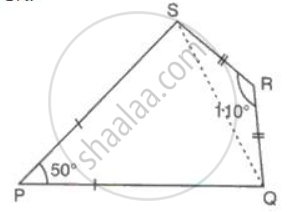Exercise 12.1 | Q 10

ΔABC is an isosceles triangle with AB = AC. Another triangle BDC is drawn with base BC = BD in such a way that BC bisects ∠B. If the measure of ∠BDC is 70°, find the measures of ∠DBC and ∠BAC.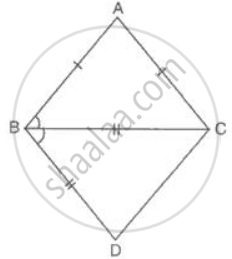Exercise 12.1 | Q 11.1

ΔPQR is isosceles with PQ = PR. T is the mid-point of QR, and TM and TN are perpendiculars on PR and PQ respectively. Prove that,
TM = TN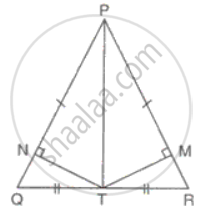Exercise 12.1 | Q 11.2

ΔPQR is isosceles with PQ = PR. T is the mid-point of QR, and TM and TN are perpendiculars on PR and PQ respectively. Prove that,
PM = PNExercise 12.1 | Q 11.3

ΔPQR is isosceles with PQ = PR. T is the mid-point of QR, and TM and TN are perpendiculars on PR and PQ respectively. Prove that,
PT is the bisector of ∠P.Exercise 12.1 | Q 12

ΔPQR is isosceles with PQ = QR. QR is extended to S so that ΔPRS becomes isosceles with PR = PS. Show that ∠PSRSP : ∠QPS = 1 : 3

Exercise 12.1 | Q 13

In ΔKLM , KT bisects ∠LKM and KT = TM. If ∠LTK is 80°, find the value of ∠LMK and ∠KLM.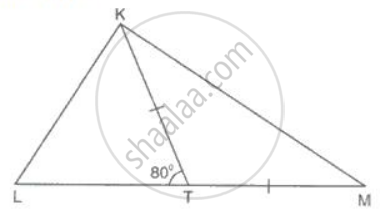Exercise 12.1 | Q 14

Equal sides QP and RP of an isosceles ΔPQR are produced beyond P to S and T such that ΔPST is an isosceles triangle with PS = PT. Prove that TQ = SR.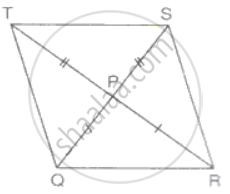Exercise 12.1 | Q 15

Prove that the bisector of the vertex angle of an isosceles triangle bisects the base perpendicularly.

Exercise 12.1 | Q 16.1

In the figure ΔABC is isosceles with AB = AC. Prove that:
∠A : ∠B = 1 : 3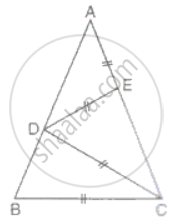Exercise 12.1 | Q 16.2

In the figure ΔABC is isosceles with AB = AC. Prove that:Exercise 12.1 | Q 17.1

In ΔABC, D is the mid-point of BC, AD is equal to AC. AC is produced to E, such that CE = AC. Prove that: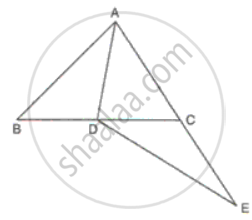Exercise 12.1 | Q 17.2

In ΔABC, D is the mid-point of BC, AD is equal to AC. AC is produced to E, such that CE = AC. Prove that:
AB = CEExercise 12.1 | Q 18

In ΔXYZ, AY and AZ are the bisector of ∠Y and ∠Z respectively. The perpendicular bisectors of AY and AZ cut YZ at B and C respectively. Prove that line segment YZ is equal to the perimeter of ΔABC.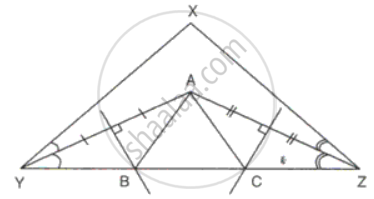Exercise 12.1 | Q 19

ΔPQR  is an isosceles triangle with PQ = PR. QR is extended to S and ST is drawn perpendicular to QP produced, and SN is perpendicular to PR produced. Prove that QS bisects ∠TSN.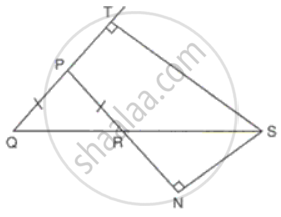Exercise 12.1 | Q 20

In the given figure, D and E are points on AB and AC respectively. AE and CD intersect at P such that AP = CP. If ∠BAE = ∠BCD, prove that DBDE is isosceles.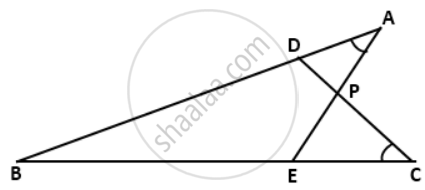Exercise 12.1 | Q 21

In DPQR as shown, ∠PQS = ∠RQS and QS ⊥ PR. Find the value of x and y, if PQ = 3x + 1; QR = 5y - 2; PS = x + 1 and SR = y + 2.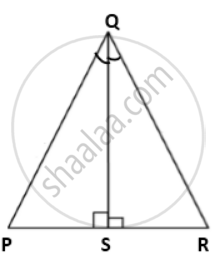Exercise 12.1 | Q 22.1

In the given figure :
if ∠PQS = 60°,
find∠QPR.

Exercise 12.1 | Q 22.2

In the given figure :
if ∠PQS = 60°,
show that PQ = PS = QS = SR.

Exercise 12.1 | Q 23

In the give figure, if DPQR is an isosceles triangle, prove that: ∠QSR = exterior ∠PRT.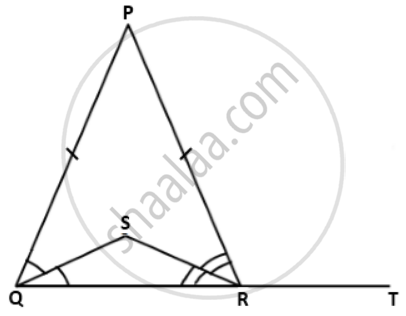Exercise 12.1 | Q 24

In the given figure, if DABC is an isosceles triangle and ∠PAC = 110o, find the base angle and vertex angle of the DABC.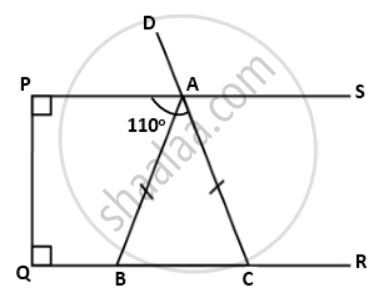## Chapter 12: Isosceles Triangle

Exercise 12.1## Frank solutions for Class 9 Maths ICSE chapter 12 - Isosceles Triangle

Frank solutions for Class 9 Maths ICSE chapter 12 (Isosceles Triangle) include all questions with solution and detail explanation. This will clear students doubts about any question and improve application skills while preparing for board exams. The detailed, step-by-step solutions will help you understand the concepts better and clear your confusions, if any. Shaalaa.com has the CISCE Class 9 Maths ICSE solutions in a manner that help students grasp basic concepts better and faster.

Further, we at Shaalaa.com provide such solutions so that students can prepare for written exams. Frank textbook solutions can be a core help for self-study and acts as a perfect self-help guidance for students.

Concepts covered in Class 9 Maths ICSE chapter 12 Isosceles Triangle are Isosceles Triangles, Isosceles Triangles : If Two Sides of a Triangle Are Equal, the Angles Opposite to Them Are Also Equal., Isosceles Triangle : If Two Angles of a Triangle Are Equal, the Sides Opposite to Them Are Also Equal..

Using Frank Class 9 solutions Isosceles Triangle exercise by students are an easy way to prepare for the exams, as they involve solutions arranged chapter-wise also page wise. The questions involved in Frank Solutions are important questions that can be asked in the final exam. Maximum students of CISCE Class 9 prefer Frank Textbook Solutions to score more in exam.

Get the free view of chapter 12 Isosceles Triangle Class 9 extra questions for Class 9 Maths ICSE and can use Shaalaa.com to keep it handy for your exam preparation